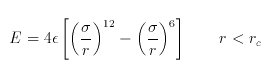SPPARKS Website - SPPARKS Documentation - SPPARKS Commands

### pair_style lj command

Syntax:

```pair_style lj Ntypes cutoff
```
• lj = style name of this pair style
• Ntypes = # of particle types
• cutoff = global cutoff for pairwise interactions (distance units)

Examples:

```pair_style lj 1 2.5
pair_style lj 3 3.0
```

Description:

The lj/cut style computes the standard 12/6 Lennard-Jones potential, given byRc is the cutoff.

The following coefficients must be defined for each pair of particle types via the pair_coeff command, or by mixing as described below:

• epsilon (energy units)
• sigma (distance units)
• cutoff (distance units)

Note that sigma is defined in the LJ formula as the zero-crossing distance for the potential, not as the energy minimum at 2^(1/6) sigma.

The last coefficients is optional. If not specified, the global LJ cutoff specified in the pair_style command is used.

Mixing info:

For atom type pairs I,J and I != J, the epsilon and sigma coefficients and cutoff distance for all of the lj/cut pair styles can be mixed. The style of mixing is geometric, which means that

```epsilon_ij = sqrt(epsilon_i * epsilon_j)
sigma_ij = sqrt(sigma_i * sigma_j)
```

Restrictions: none

Related commands: none

Default: none